# Hundreds Chart PrintableMay 07, 2021By Printablee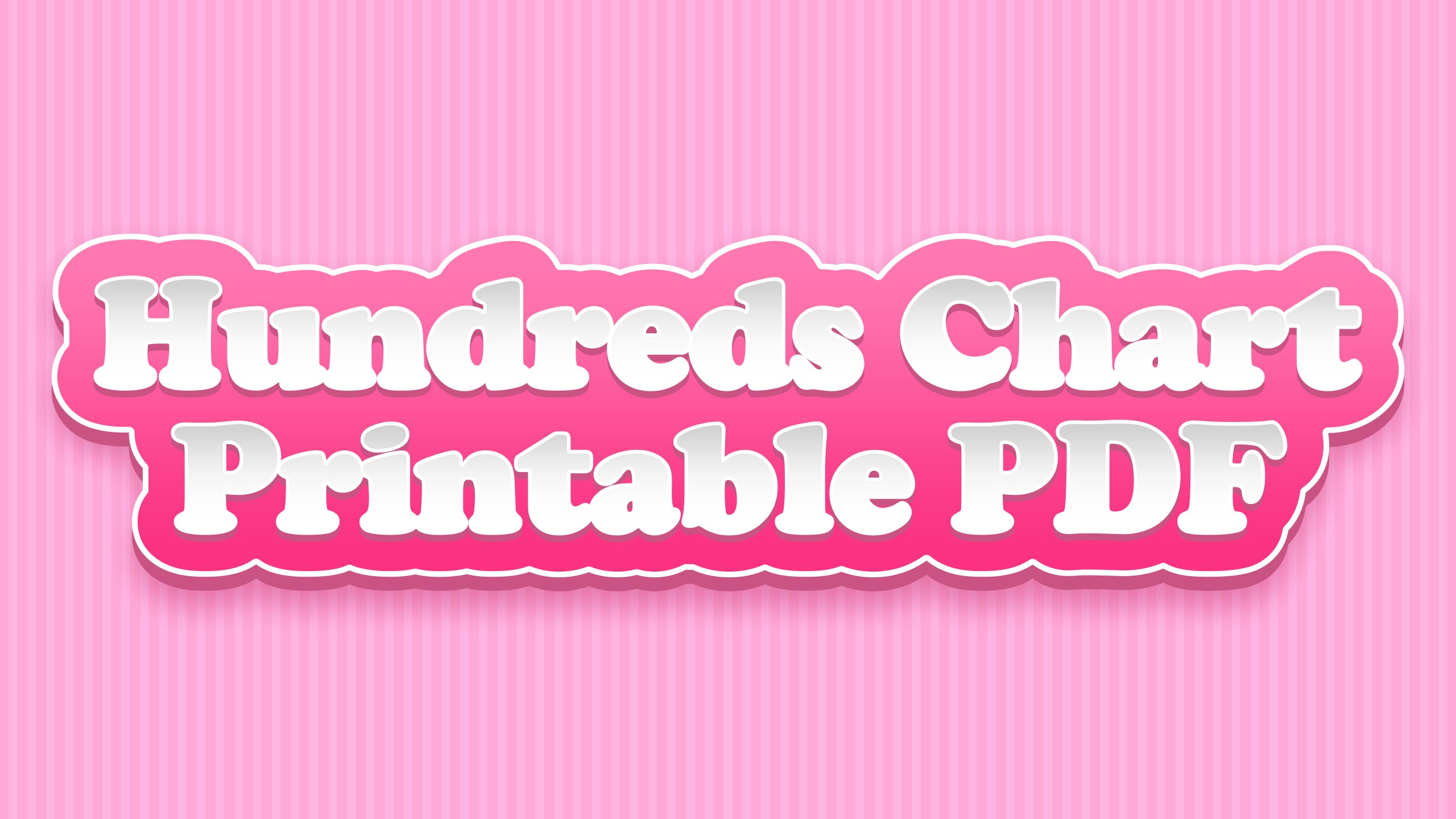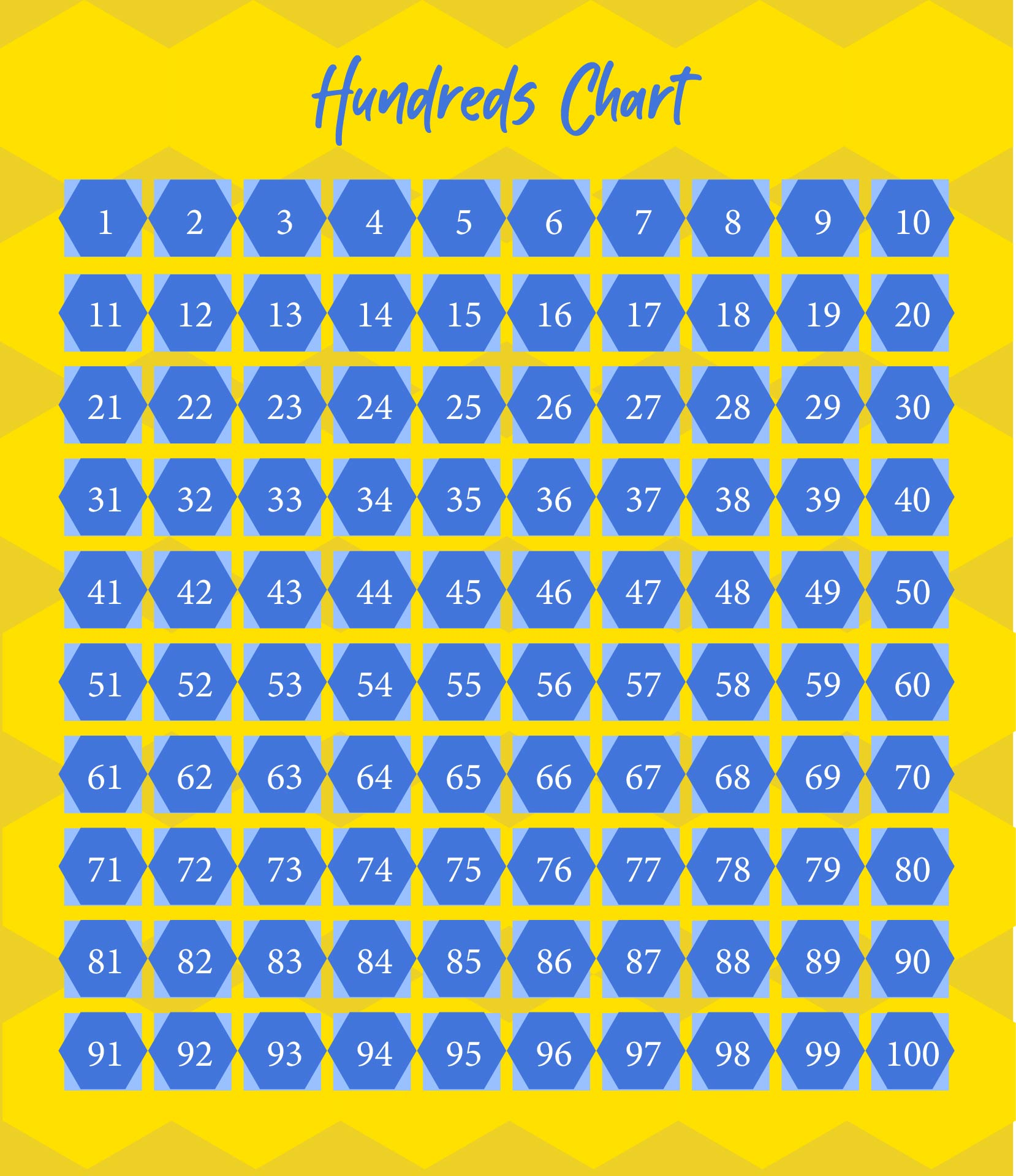## What is a Number Chart?

Number chart is a table that lists various numbers in numerical order. The order starts from 1-10 for the first row, 11-20 for the second row, 21-30 for the third row, and so on. Number charts have many different types of charts, including hundred charts with the numbers 1-100.

Children need to understand number charts. That's because number charts can help children to memorize number calculations more easily. In addition, children can also identify various numbers and numerical sequences. Number charts can also help children to recognize and analyze patterns in numbers. Examples are odd, even, tens, hundreds, and so on.

Some types of number charts have both written numbers and integers. It is combined with pictures of several objects with the number corresponding to the written number. Then, what are the types of number charts?

• Hundred Chart: The most common and widely used type of number chart is the hundred chart. The numbers written on the chart are 1-100.
• Number Chart in Words: The second type of number chart is the number chart in words. So, there are numbers and writing on the chart. This is a number chart that can be used to make it easier for children to read numbers.
• Number Chart 500-1000: There is also a number chart 500-1000 which contains the numbers from 500 to 1000.
• Number Chart Patterns: Another type of number chart is number chart patterns. There are several types of number chart patterns such as even number patterns, odd number patterns, and others. Usually, children are asked to fill in the blanks of the number chart patterns.

## What is the Hundred Chart?

After understanding the explanation of the number chart above, you must already understand the various types of number charts. One of them is the hundred chart which is a type of number chart. The hundred chart is the best tool used to train elementary school children's math skills.

There is a 10x10 grid on the hundred chart. Although it is used to understand 100 numbers, it is also used to understand number values and patterns.

Hundred charts are usually used to perform calculations of up to 100 numbers in various ways. An example is counting the numbers one by one in a row or diagonally. Therefore, a hundred charts are very good to be implemented in learning mathematics and games.

## How Do Number Chart and Hundred Chart Help Children to Count?

As explained above, the hundred chart is part of the number chart, so both of them have the same function in helping children learn to count. Therefore, let's discuss more deeply about number charts vs hundred charts: how do the charts help children count?

So, the number chart and hundred charts can help children learn to count through the patterns in the chart. A hundred charts and number charts have some blanks to fill in. Well, that empty area can be filled with simple mathematical calculations. Therefore, by regularly filling in the number chart or hundred chart worksheet, children can have good numeracy skills.

To make children can calculate it more easily, parents need to help children explain how to calculate using the hundred chart or number chart. Use simple worksheets that are easy for children to understand. If they are experts at doing the calculations there, start looking for a number chart or another hundred charts which is more difficult.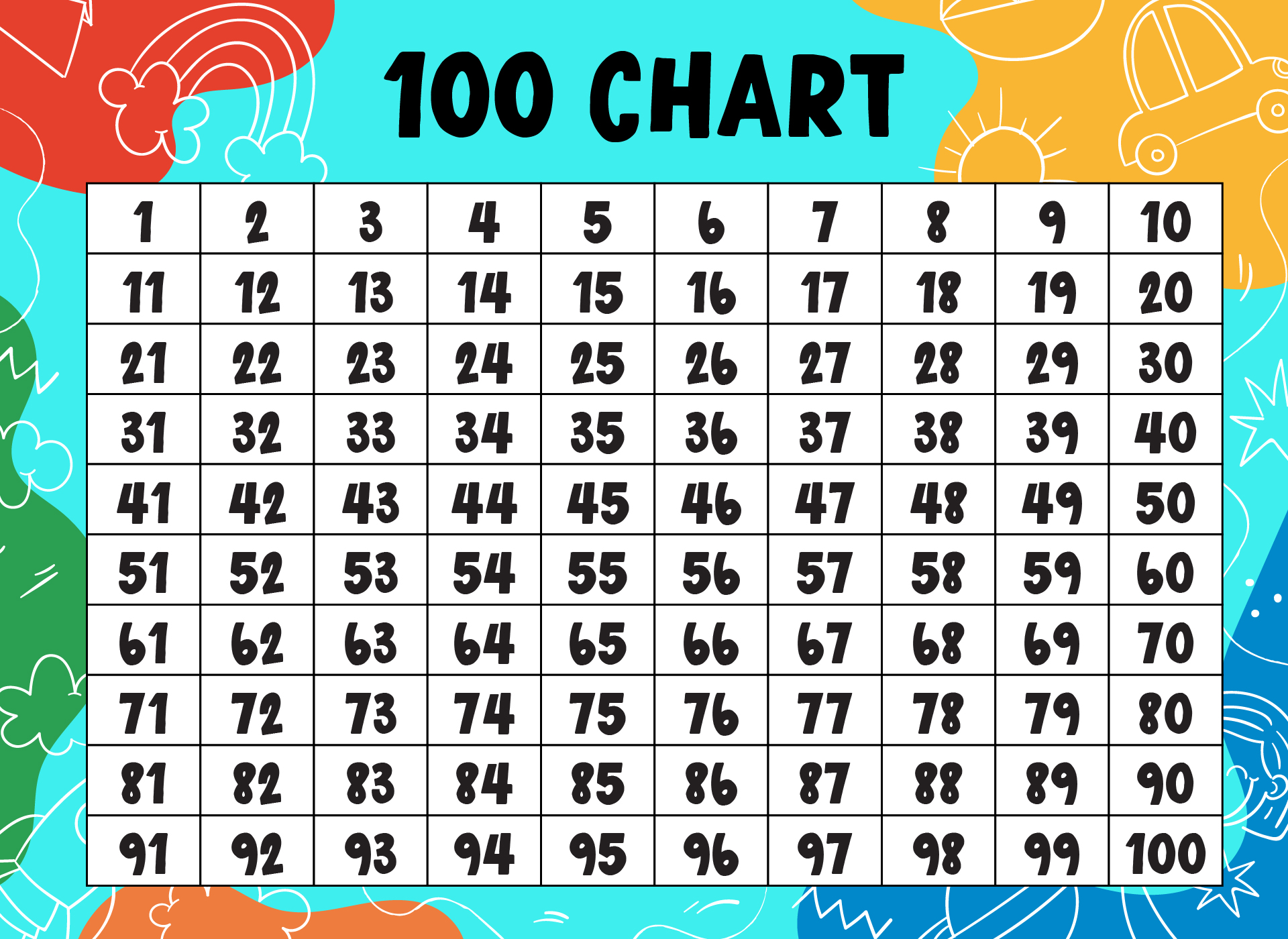We also have more printable chart you may like:
Full Size Printable 100 Chart
100 Chart Full Page Printable
100 Number Grid Chart Printable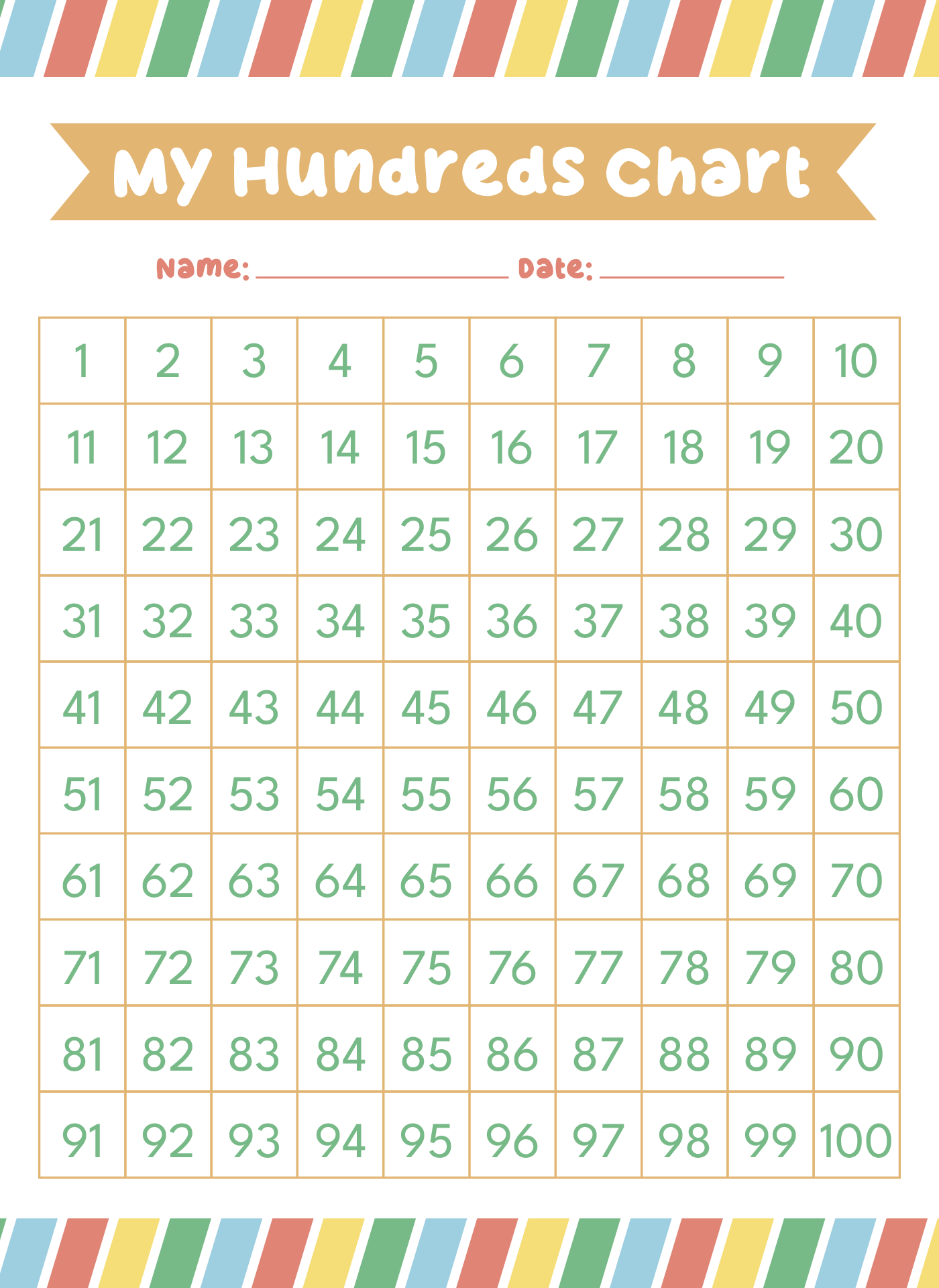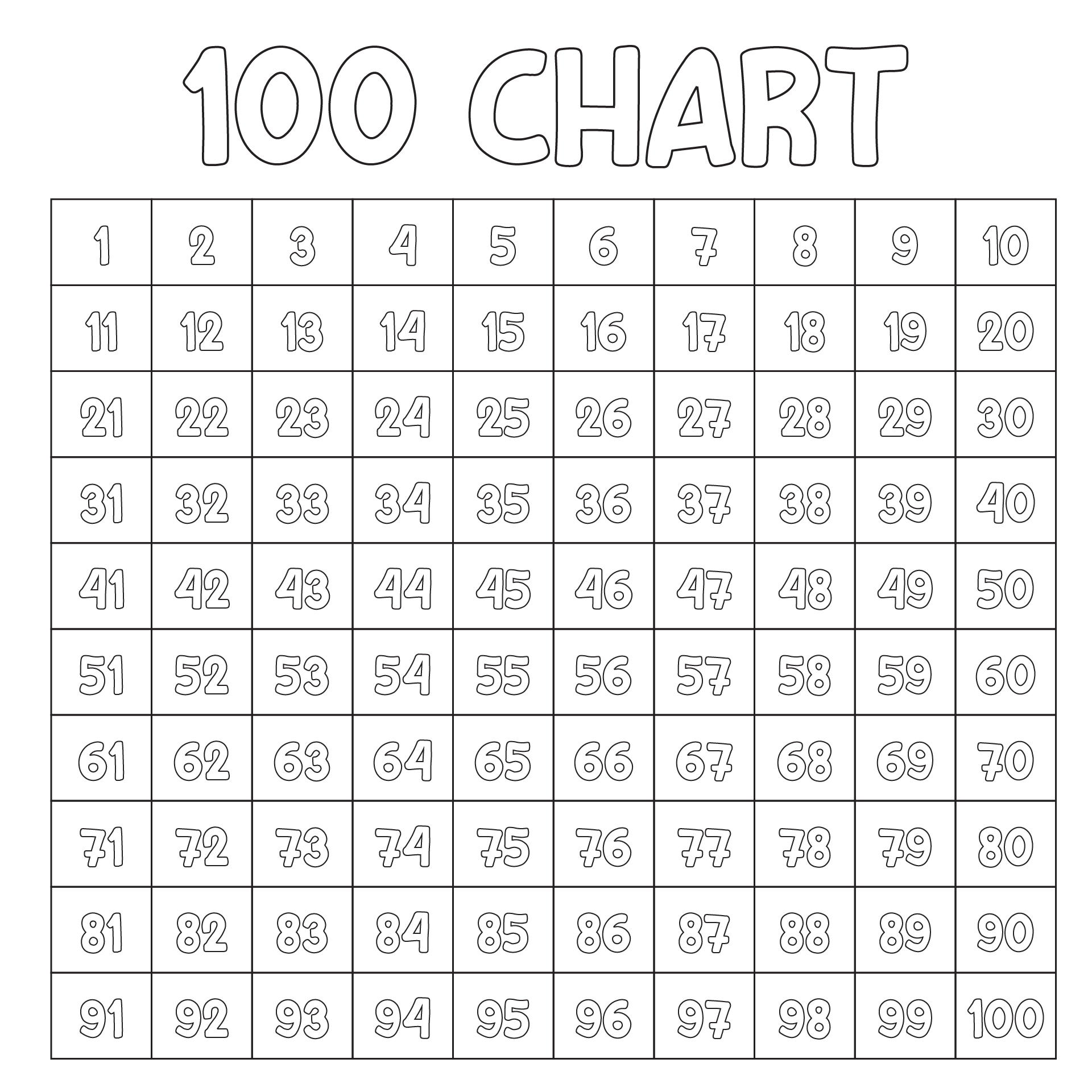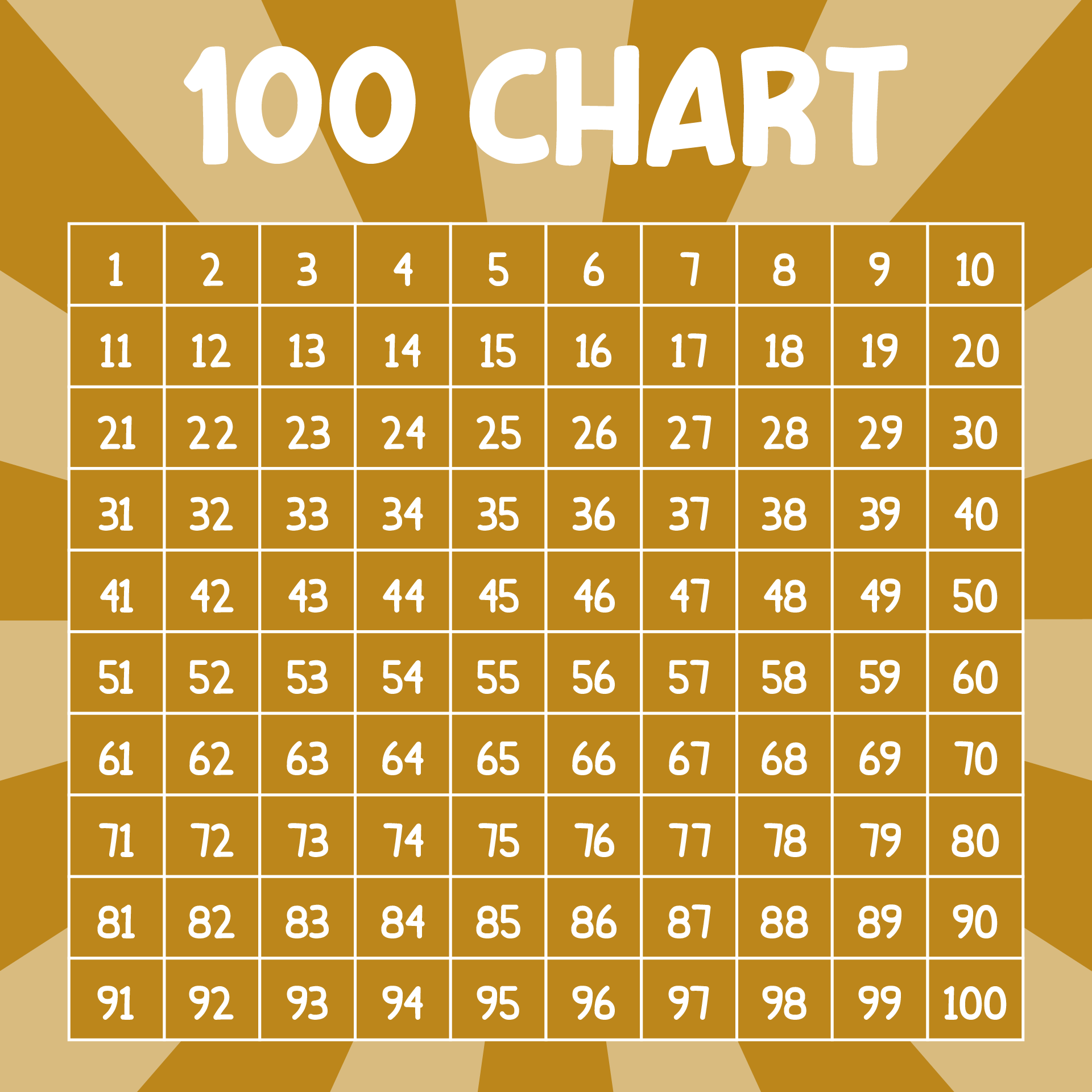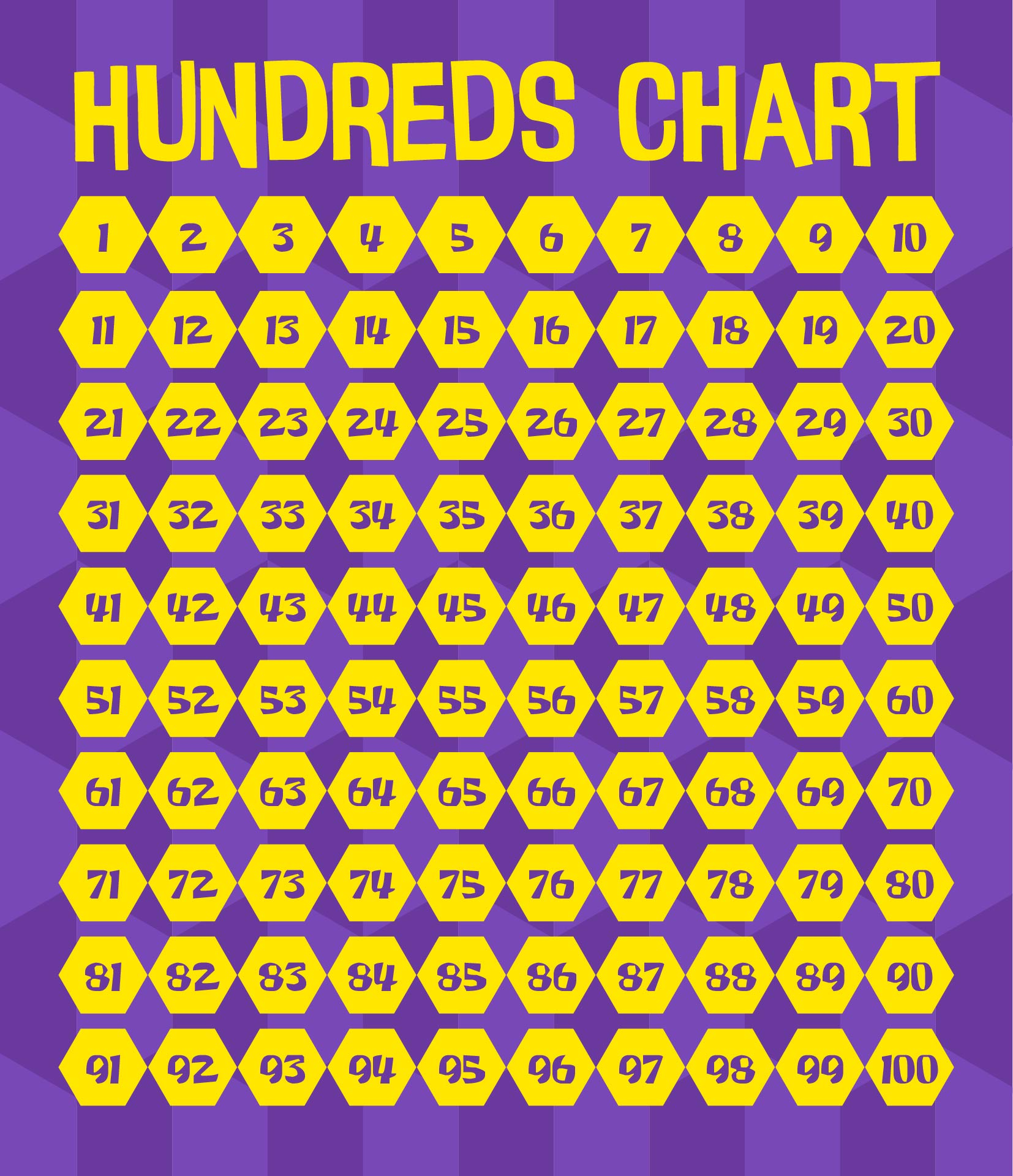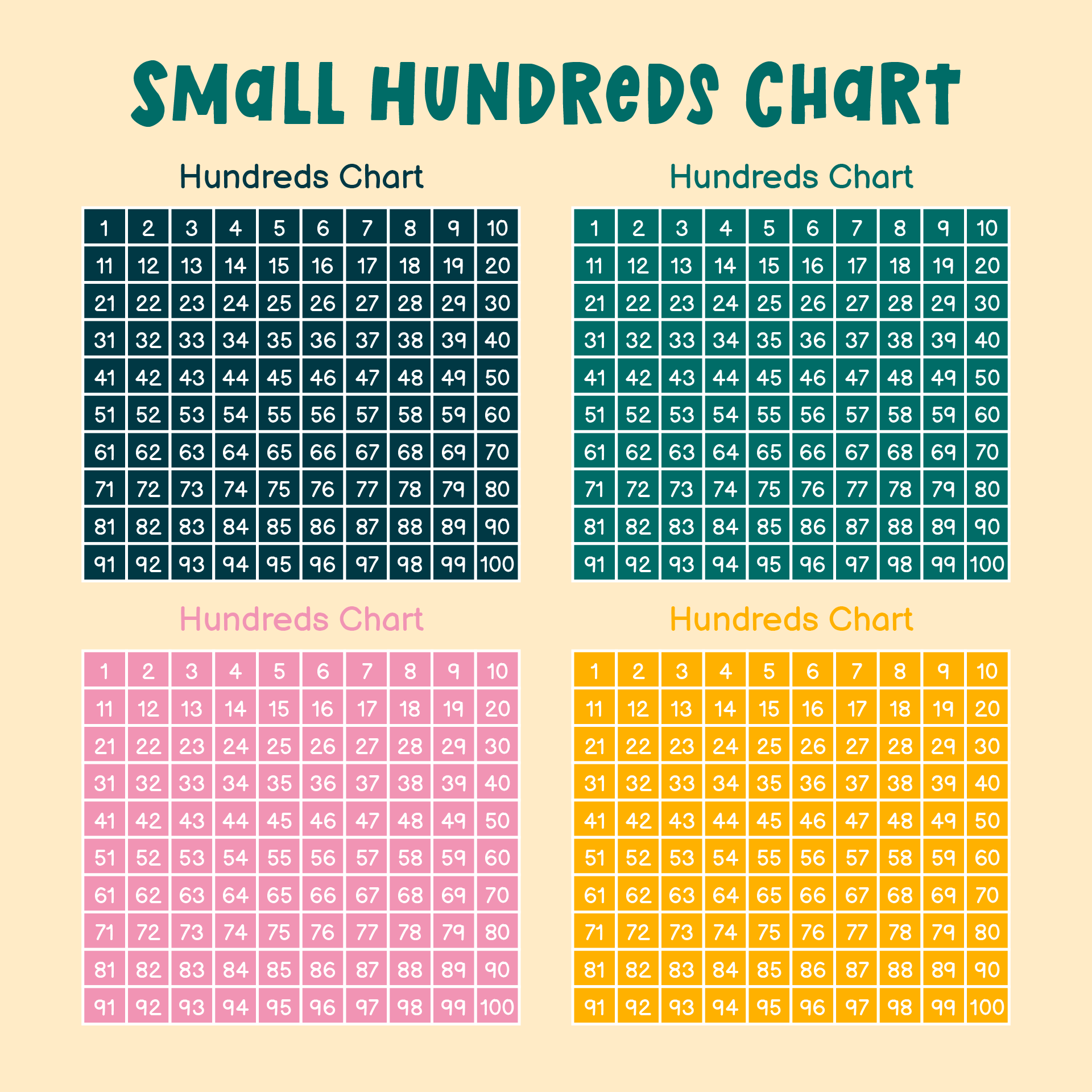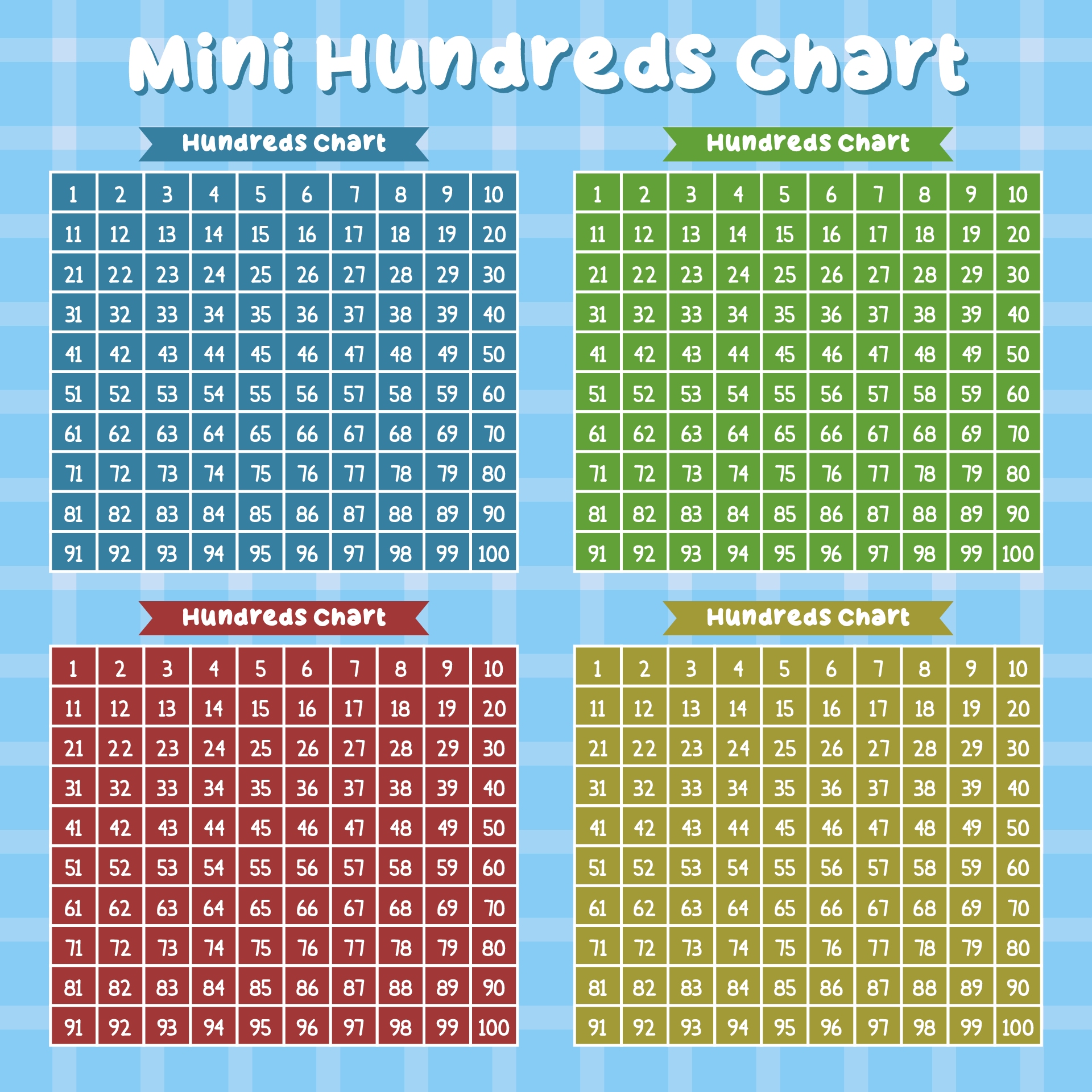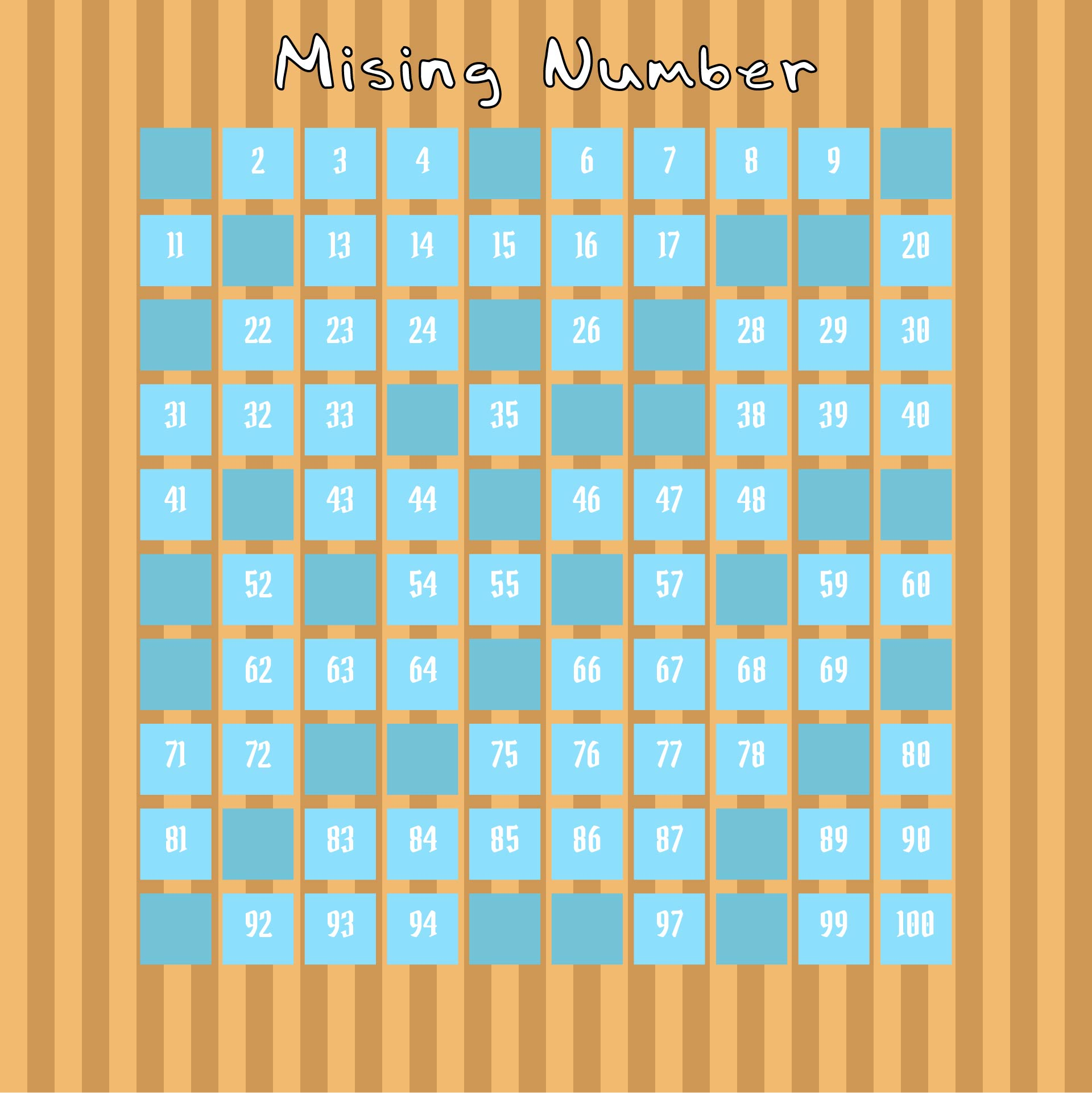## How to Teach Kids to Get Good Skills at Counting?

Number charts and hundred charts are closely related to the concept of mathematical calculations. Therefore, teaching these two types of charts is very necessary to do.

However, what if the children have difficulty doing calculations using these charts? This is the role of parents. You can help children learn to count through the number charts and hundred charts by applying these tips.

• The easiest counting method is to use fingers. This is also a way of counting that has been done since centuries ago. So, you can apply this method to teach children to count easily using a hundred charts or number charts. In fact, this method is highly recommended by the National Association for the Education of Young Children (NAEYC). By learning to count using their fingers, children can easily learn to use other calculation tools.

• You can teach children to count under certain conditions. Examples are when tidying up toys, shopping, and walking outside the house. This is a basic way to make it easier for children to do calculations and recognize numbers on number charts or hundred charts.

• If you want to use a more fun way, you can ask the children to role-play. Let them assume a certain number-related role. An example is acting as a trader.

• Children can also learn the number chart and hundred chart while playing in nature. This is one of the children's learning innovations. By learning outdoors, children won't be bored and can get new or more fun vibes.

• You can also help the children count through songs. This is an easy way to teach preschool and kindergarten kids about numbers and counting. With songs, children will find it easier to understand and recognize various numbers.

• Don't forget to appreciate the children when they manage to say the numbers and do the calculations correctly. With support from parents, children will be more confident in developing their numeracy skills.